# 2018 CSAT (Korean SAT) EBS mock test (Natural Sciences, Grade 12)

.

$\large \text{2018 academic year College Scholastic Ability Test EBS mock test (version 1)}$

$\Huge \text{Math Section} ~ \Large \text{(Natural Sciences)}$

.

$\huge \text{Rules:}$

1. This post is for people who really want to experience how Korean SAT works.

2. This test has a time limit of 100 minutes.

3. Any use of calculators is considered cheating.

4. This test consists of 30 questions.

5. The first 21 are multiple choice questions, and the rest, 9, are short-answer questions.

6. The total score is 100 points.

7. The amount of points alloted to each question is marked at the end of the question, so keep that in mind.

9. If you don't have enough time to finish those all, then try #20, #21, #28, #29, #30. Those are the hardest ones among all.

10. Have fun!

.

.

.

.

.

$\large \boxed{\text{Multiple choice}}$

$\huge 1.~$ There are two vectors $\vec{a}=(1,~2),$ and $\vec{b}=(2,~-3).$ Find the sum of the components of the vector $\vec{a}+2\vec{b}.$ $~$ [2 points]

\begin{aligned}&\boxed{\text{A}}. && 1 \\\\ &\boxed{\text{B}}. && 2 \\\\ &\boxed{\text{C}}. && 3 \\\\ &\boxed{\text{D}}. && 4 \\\\ &\boxed{\text{E}}. && 5 \end{aligned}

.

.

.

$\huge 2.~$ What's the value of $\displaystyle \lim_{x\to0}\frac{e^{3x}-1}{2x^2+3x}?$ $~$ [2 points]

\begin{aligned}&\boxed{\text{A}}. && \frac{1}{3} \\\\ &\boxed{\text{B}}. && \frac{1}{2} \\\\ &\boxed{\text{C}}. && 1 \\\\ &\boxed{\text{D}}. && 2 \\\\ &\boxed{\text{E}}. && 3 \end{aligned}

.

.

.

$\huge 3.~$ Find the value of $\displaystyle \int_{0}^{1} 5x\sqrt{x}dx.$ $~$ [2 points]

\begin{aligned}&\boxed{\text{A}}. && 2 \\\\ &\boxed{\text{B}}. && 4 \\\\ &\boxed{\text{C}}. && 6 \\\\ &\boxed{\text{D}}. && 8 \\\\ &\boxed{\text{E}}. && 10 \end{aligned}

.

.

.

$\huge 4.~$ $X^C$ denotes the complementary event of an event $X.$ Two events $A$ and $B$ are independent, and $\mathrm{P}(A^C)=\dfrac{1}{3},~\mathrm{P}(A\cap B)=\dfrac{1}{6}.$ Find the value of $\mathrm{P}(A\cup B).$ $~$ [3 points]

\begin{aligned}&\boxed{\text{A}}. && \frac{1}{2} \\\\ &\boxed{\text{B}}. && \frac{2}{3} \\\\ &\boxed{\text{C}}. && \frac{3}{4} \\\\ &\boxed{\text{D}}. && \frac{4}{5} \\\\ &\boxed{\text{E}}. && \frac{5}{6} \end{aligned}

.

.

.

$\huge 5.~$ How many odd, 3-digit natural numbers are there whose digits only consist of 1, 2, 3, 4, 5? $~$ [3 points]

\begin{aligned}&\boxed{\text{A}}. && 55 \\\\ &\boxed{\text{B}}. && 60 \\\\ &\boxed{\text{C}}. && 65 \\\\ &\boxed{\text{D}}. && 70 \\\\ &\boxed{\text{E}}. && 75 \end{aligned}

.

.

.

$\huge 6.~$ Let $g(x)$ be the inverse function of $f(x)=e^{x^3+1}.$ What's the value of $g'(1)?$ $~$ [3 points]

\begin{aligned}&\boxed{\text{A}}. && \frac{1}{3} \\\\ &\boxed{\text{B}}. && \frac{1}{2} \\\\ &\boxed{\text{C}}. && 1 \\\\ &\boxed{\text{D}}. && 2 \\\\ &\boxed{\text{E}}. && 3 \end{aligned}

.

.

.

$\huge 7.~$ When throwing a dice 3 times, find the probability of the product of the three results being a multiple of 9. $~$ [3 points]

\begin{aligned}&\boxed{\text{A}}. && \frac{2}{27} \\\\ &\boxed{\text{B}}. && \frac{1}{9} \\\\ &\boxed{\text{C}}. && \frac{4}{27} \\\\ &\boxed{\text{D}}. && \frac{2}{9} \\\\ &\boxed{\text{E}}. && \frac{7}{27} \end{aligned}

.

.

.

$\huge 8.~$ There are two points $\mathrm{A}(1,~2,~3),~\mathrm{B}(-2,~5,~-3)$ in a coordinate space. A point $\mathrm{P}(a,~b,~c)$ is on segment $\mathrm{AB},$ satisfying $\overline{\mathrm{AP}}=2\overline{\mathrm{PB}}.$ Find the value of $a+b+c.$ $~$ [3 points]

\begin{aligned}&\boxed{\text{A}}. && -2 \\\\ &\boxed{\text{B}}. && -1 \\\\ &\boxed{\text{C}}. && 0 \\\\ &\boxed{\text{D}}. && 1 \\\\ &\boxed{\text{E}}. && 2 \end{aligned}

.

.

.

$\huge 9.~$ What's the value of $\displaystyle \int_{1}^{e} \ln(x^2)dx?$ $~$ [3 points]

\begin{aligned}&\boxed{\text{A}}. && 1 \\\\ &\boxed{\text{B}}. && 2 \\\\ &\boxed{\text{C}}. && 3 \\\\ &\boxed{\text{D}}. && 4 \\\\ &\boxed{\text{E}}. && 5 \end{aligned}

.

.

.

$\huge 10.~$ The position of a moving point $\mathrm{P}(x,~y)$ at time $t~(t>0)$ is $\cases{x=2\sqrt{t} \\\\ y=\dfrac{4}{t+1}}.$ What is the speed at which $\mathrm{P}$ moves at $t=1?$ $~$ [3 points]

\begin{aligned}&\boxed{\text{A}}. && 1 \\\\ &\boxed{\text{B}}. && \sqrt{2} \\\\ &\boxed{\text{C}}. && \sqrt{3} \\\\ &\boxed{\text{D}}. && 2 \\\\ &\boxed{\text{E}}. && \sqrt{5} \end{aligned}

.

.

.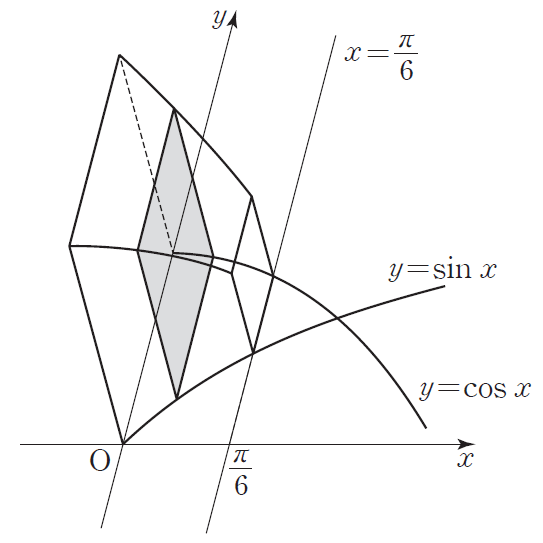$\huge 11.~$ As shown on the right, there is a solid figure whose base side is the area enclosed by two curves $y=\sin x,~y=\cos x,$ the $y$-axis and a line $x=\dfrac{\pi}{6}.$ Every longitudinal section perpendicular to the $x$-axis is a square. What's the volume of this solid? $~$ [3 points]

\begin{aligned}&\boxed{\text{A}}. && \frac{2\pi - 1}{12} \\\\ &\boxed{\text{B}}. && \frac{2\pi - 3}{12} \\\\ &\boxed{\text{C}}. && \frac{2\pi - 5}{12} \\\\ &\boxed{\text{D}}. && \frac{4\pi - 7}{12} \\\\ &\boxed{\text{E}}. && \frac{4\pi - 9}{12} \end{aligned}

.

.

.

$\huge 12.~$ $\theta$ is the acute angle that line $x=2y+1=z+3$ and the $x$-axis form, in a coordinate space. What's the value of $\cos \theta ?$ $~$ [3 points]

\begin{aligned}&\boxed{\text{A}}. && \frac{1}{6} \\\\ &\boxed{\text{B}}. && \frac{1}{3} \\\\ &\boxed{\text{C}}. && \frac{1}{2} \\\\ &\boxed{\text{D}}. && \frac{2}{3} \\\\ &\boxed{\text{E}}. && \frac{5}{6} \end{aligned}

.

.

.

$\huge 13.~$ A sample of size $16$ and average $\overline{X}$ is arbitrarily chosen from a population that follows the normal distribution $\mathrm{N}(m,~1).$ The random variable of the population is $X.$ Given that $\mathrm{P}(X\ge5)=\mathrm{P}(\overline{X}\le 1)$ and $m<5,$ find the value of $m.$ $~$ [3 points]

\begin{aligned}&\boxed{\text{A}}. && \frac{3}{5} \\\\ &\boxed{\text{B}}. && 1 \\\\ &\boxed{\text{C}}. && \frac{7}{5} \\\\ &\boxed{\text{D}}. && \frac{9}{5} \\\\ &\boxed{\text{E}}. && \frac{11}{5} \end{aligned}

.

.

.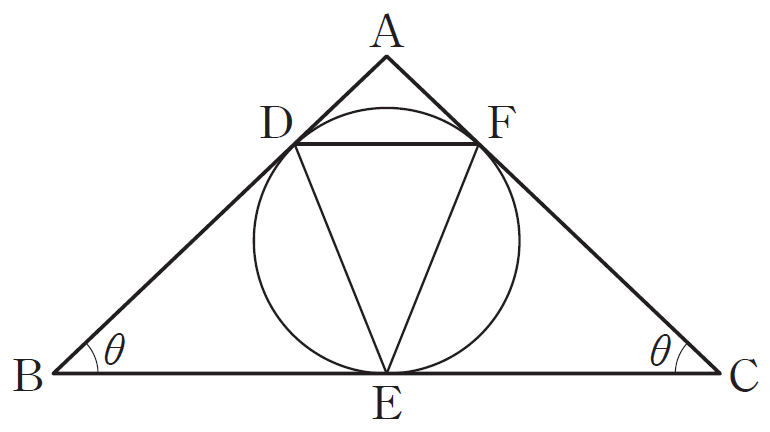$\huge 14.~$ As shown on the right, let $\mathrm{D},~\mathrm{E},~\mathrm{F}$ be the point of contact between the inscribed circle of triangle $\mathrm{ABC}$ and $\overline{\mathrm{AB}},~\overline{\mathrm{BC}},~\overline{\mathrm{CA}},$ where $\angle \mathrm{ABC}=\theta,~\angle \mathrm{BCA}=\theta,~ \overline{\mathrm{BC}}=2.$ Define $S(\theta)$ as the area of triangle $\mathrm{DEF}.$ Find the value of $\displaystyle \lim_{\theta\to 0^+ }\frac{S(\theta)}{\theta^3}.$ $~$ [4 points]

\begin{aligned}&\boxed{\text{A}}. && \frac{1}{4} \\\\ &\boxed{\text{B}}. && \frac{1}{2} \\\\ &\boxed{\text{C}}. && 1 \\\\ &\boxed{\text{D}}. && 2 \\\\ &\boxed{\text{E}}. && 4 \end{aligned}

.

.

.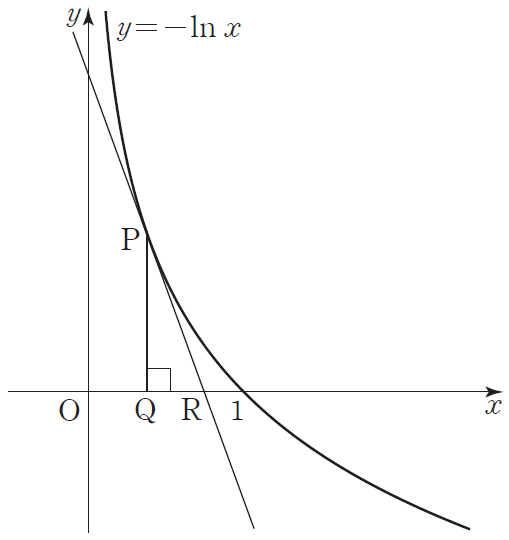$\huge 15.~$ There's a point $\mathrm{P}(t,~-\ln t)~(0 on the curve $y=-\ln x.$ $\mathrm{Q}$ is the foot of perpendicular from $\mathrm{P}$ to the $x$-axis, and $\mathrm{R}$ is the point of intersection of the tangent line from $\mathrm{P}$ and the $x$-axis. What's the maximum area of triangle $\mathrm{PQR}?$ $~$ [4 points]

\begin{aligned}&\boxed{\text{A}}. && \frac{1}{e^2} \\\\ &\boxed{\text{B}}. && \frac{2}{e^2} \\\\ &\boxed{\text{C}}. && \frac{3}{e^2} \\\\ &\boxed{\text{D}}. && \frac{4}{e^2} \\\\ &\boxed{\text{E}}. && \frac{5}{e^2} \end{aligned}

.

.

.

$\huge 16.~$ Two fixed points, $\mathrm{A},~\mathrm{B},$ satisfy $\overline{\mathrm{AB}}=2.$ A point $\mathrm{P}$ satisfies the below conditions.

(1) $\overrightarrow{\mathrm{AP}}\cdot\overrightarrow{\mathrm{PB}}=0.$

(2) $\overrightarrow{\mathrm{AB}}\cdot\overrightarrow{\mathrm{AP}}\ge2+\sqrt{3}$

What is the length of the locus of $\mathrm{P}?$ $~$ [4 points]

\begin{aligned}&\boxed{\text{A}}. && \frac{\pi}{3} \\\\ &\boxed{\text{B}}. && \frac{\pi}{2} \\\\ &\boxed{\text{C}}. && \frac{2\pi}{3} \\\\ &\boxed{\text{D}}. && \frac{5\pi}{6} \\\\ &\boxed{\text{E}}. && \pi \end{aligned}

.

.

.

$\huge 17.~$ For a natural number $n\ge2,$ there's a pocket that contains $n$ cards where each card is written a different natural number from $1$ to $n.$ Choose 2 cards randomly, at the same time, from the pocket. Let the random variable $X$ be the product of the two numbers written on them. Below is the process of finding $E(X).$

When choosing 2 cards randomly, at the same time, from $n$ cards, the probability of choosing $2$ particular cards is $\dfrac{2}{\boxed{\text{ (a) }}}.$

Therefore, if we let $S$ be the sum of all product of the two chosen cards, $E(X)=\dfrac{2}{\boxed{\text{ (a) }}}\times S.$

Meanwhile, if we let $r~(2\ge r \ge n)$ be the bigger number between the two chosen numbers,

\begin{aligned} S & = \sum_{r=2}^{n} \left[r\times\{1+2+3+ \cdots +(r-2)+(r-1)\}\right] \\ & =\sum_{r=2}^{n} \left\{r\times \frac{r(r-1)}{2}\right\} \\ & = \frac{1}{2}\sum_{r=2}^{n} (r^3-r^2) \\ & = \frac{1}{2}\sum_{r=1}^{n} (r^3-r^2) \\ & = \frac{n(n+1)(n-1)(\boxed{\text{ (b) }})}{24}\end{aligned}

To sum up,

$E(X)=\dfrac{\boxed{\text{ (c) }}}{12}.$

The correct expressions that fit in the blanks $\text{(a), (b), (c)}$ are $f(n),~g(n),~h(n),$ respectively. Find the value of $f(2)+g(3)+h(4).$ $~$ [4 points]

\begin{aligned}&\boxed{\text{A}}. && 80 \\\\ &\boxed{\text{B}}. && 81 \\\\ &\boxed{\text{C}}. && 82 \\\\ &\boxed{\text{D}}. && 83 \\\\ &\boxed{\text{E}}. && 84 \end{aligned}

.

.

.

$\huge 18.~$ A random variable $X$ that follows the normal distribution and a random variable $Z$ that follows the standard normal distribution satisfy the below conditions for some constant $a.$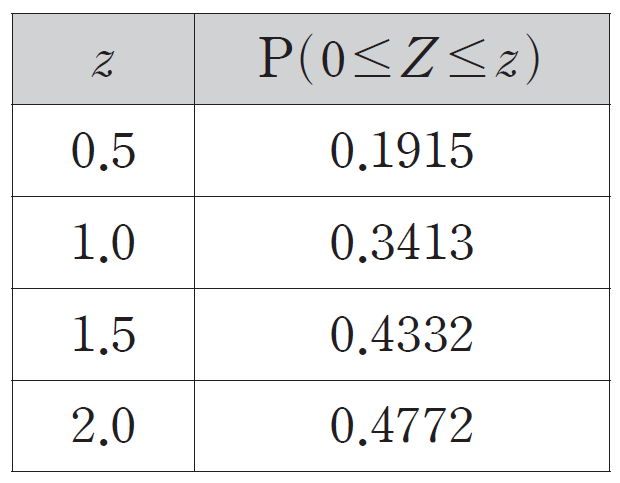(1) $X = a+2Z$

(2) $\mathrm{P}(X\le 1)=\mathrm{P}(X\ge 5)$

Find the value of $\mathrm{P}(a-2\le X \le 2a+1)$ using the standard normal distribution table shown on the right. $~$ [4 points]

\begin{aligned}&\boxed{\text{A}}. && 0.5328 \\\\ &\boxed{\text{B}}. && 0.6687 \\\\ &\boxed{\text{C}}. && 0.7745 \\\\ &\boxed{\text{D}}. && 0.8185 \\\\ &\boxed{\text{E}}. && 0.9104 \end{aligned}

.

.

.

$\huge 19.~$ A parabola $y^2=4x$ has $\mathrm{F}$ as its focus, and some point in the first quadrant, $\mathrm{P},$ is on the parabola satisfying $\overline{\mathrm{PF}}=4.$ Let $\mathrm{Q}$ be where the tangent line from $\mathrm{P}$ intersects the $x$-axis, and let $\mathrm{R}$ be where that tangent line intersects the circle with $\overline{\mathrm{QF}}$ as its radius. Find the area of triangle $\mathrm{FRQ}.$ ($\rm R\neq Q$) $~$ [4 points]

\begin{aligned}&\boxed{\text{A}}. && 2\sqrt{3} \\\\ &\boxed{\text{B}}. && 3\sqrt{2} \\\\ &\boxed{\text{C}}. && 3\sqrt{3} \\\\ &\boxed{\text{D}}. && 4\sqrt{2} \\\\ &\boxed{\text{E}}. && 4\sqrt{3} \end{aligned}

.

.

.

$\huge 20.~$ For a function $f(x)=(e^x-1)\cos\left(\dfrac{\pi}{2}x\right)+\dfrac{\pi}{2}x,$ which of the followings are true? $~$ [4 points]

(a) $f(1)>0$

(b) There exists a value for $a$ in the open interval $(0,~1)$ that satisfies $f'(a)>0.$

(c) There exists a value for $b$ in the open interval $(0,~1)$ that satisfies $f'(b)=0.$

\begin{aligned}&\boxed{\text{A}}. && \text{(a)} \\\\ &\boxed{\text{B}}. && \text{(c)} \\\\ &\boxed{\text{C}}. && \text{(a), (b)} \\\\ &\boxed{\text{D}}. && \text{(b), (c)} \\\\ &\boxed{\text{E}}. && \text{(a), (b), (c)} \end{aligned}

.

.

.

$\huge 21.~$ For a function $f(x)$ that's continuous in closed interval $\left[0,~1\right],$ define $\displaystyle F(x)=\int_{0}^{x}f(t)dt~(0\le x \le 1).$ These two functions satisfy the below conditions.

(1) Function $f(x)$ increases in closed interval $\left[0,~1\right],$ and $\displaystyle \int_{0}^{1}\{f(x)+|f(x)|\}dx=6.$

(2) Function $F(x)$ has its local minimum $-1$ at $x=a~(0

What's the value of $\displaystyle \int_{a}^{1}F(x)f(x)dx?$ $~$ [4 points]

\begin{aligned}&\boxed{\text{A}}. && \frac{1}{2} \\\\ &\boxed{\text{B}}. && 1 \\\\ &\boxed{\text{C}}. && \frac{3}{2} \\\\ &\boxed{\text{D}}. && 2 \\\\ &\boxed{\text{E}}. && \frac{5}{2} \end{aligned}

.

.

.

$\large \boxed{\text{Short-answer}}$

$\huge 22.~$ In the expansion of $(x+1)^7$, find the coefficient of the $x^3$ term. $~$ [3 points]

.

.

.

.

$\huge 23.~$ Find the sum of all the values for positive integer $x$ that satisfies the inequality $\left(\dfrac{1}{2}\right)^{x-5}\ge \log_{3} 81.$ $~$ [3 points]

.

.

.

.

$\huge 24.~$ The circle made by plane $x+y+z-k=0$ and sphere $x^2+y^2+z^2-2x-3=0$ intersecting has a radius of $1.$ Find the sum of all the values for real number $k.$ $~$ [3 points]

.

.

.

.

$\huge 25.~$ The sum of the $x$-coordinate of all the points that two functions $y=3\tan x$ and $y=2\cos x$ meet at $0 is $a\pi.$ Find the value of a constant $a.$ $~$ [3 points]

.

.

.

.

$\huge 26.~$ Five natural numbers $a,~b,~c,~d,~e$ satisfy $(a+b+c)(d+e)^2=36.$ How many ordered pairs $(a,~b,~c,~d,~e)$ are there? $~$ [4 points]

.

.

.

.

$\huge 27.~$ Pocket $\mathrm{A}$ and $\mathrm{B}$ both contain $4$ balls numbered from $1$ to $4.$ Amy randomly chooses $2$ balls from pocket $\mathrm{A}$ and Bob randomly chooses $2$ balls from pocket $\mathrm{B}$ at the same time. The probability of the biggest number among the chosen numbers coming only from among the balls Bob chose is $\dfrac{p}{q}.$ Find the value of $p+q.$ ($p$ and $q$ are coprime natural numbers.) $~$ [4 points]

.

.

.

.

$\huge 28.~$ Let $\mathrm{F}_1,~\mathrm{F}_1 '$ be the two foci of an ellipse $\dfrac{x^2}{a^2}+\dfrac{y^2}{b^2}=1~(a>b>0)$ and let $\mathrm{F}_2,~ \mathrm{F}_2 '$ be the two foci of a hyperbola $\dfrac{x^2}{a^2}-\dfrac{y^2}{b^2}=1.$ Then, define $\mathrm{P}_1$ as the point where the circle that is centered at $\mathrm{F}_1$ and passes through the origin intersects with the ellipse, and define $\mathrm{P}_2$ as the point where the circle that is centered at $\mathrm{F}_2$ and passes through the origin intersects with the hyperbola. The ellipse and the hyperbola satisfy the below conditions.

(1) The asymptotes of the hyperbola are $y=\pm\dfrac{\sqrt{15}}{5}x.$

(2) $\overline{\mathrm{P}_1\mathrm{F}_1}+\overline{\mathrm{P}_2\mathrm{F}_2}=9.$

Given that two points $\mathrm{P}_1$ and $\mathrm{P}_2$ are in the first quadrant, the $x$-coordinates of $\mathrm{F}_1$ and $\mathrm{F}_2$ are positive, $\mathrm{O}$ is the origin, and $\overline{\mathrm{OF}_1}>\dfrac{a}{2},$ find the value of $\overline{\mathrm{P}_2\mathrm{F}_2 '}-\overline{\mathrm{P}_1\mathrm{F}_1 '}.$ $~$ [4 points]

.

.

.

.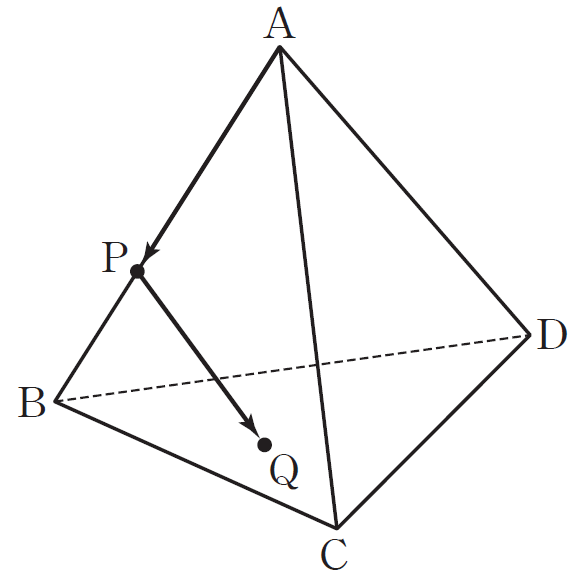$\huge 29.~$ There's a regular tetrahedron $\mathrm{ABCD}$ with side length $2.$ Let $\mathrm{P}$ be the point that divides $\overline{\mathrm{AB}}$ internally with a ratio of $2:1,$ and let $\mathrm{Q}$ be a point on the triangle $\mathrm{BCD}.$ Given that two vectors $\overrightarrow{\mathrm{AP}}$ and $\overrightarrow{\mathrm{PQ}}$ are perpendicular, the maximum of $|\overrightarrow{\mathrm{PQ}}|$ is $M,$ and $M^2=\dfrac{p}{q},$ for some coprime positive integer $p$ and $q.$ Find the value of $pq.$ $~$ [4 points]

.

.

.

.

.

$\huge 30.~$ For a 4th degree polynomial $f(x)$ whose leading coefficient is $1,$ define a function $g(x)$ whose domain is the set of all positive reals as $g(x)=\ln \dfrac{f(x)}{x}.$ Then, $g(x)$ satisfies the below condition.

Function $g(x)$ has its minima of $0$ at both $x=1$ and $x=2.$

Given that all the function values of $f(x)$ are positive, find the value of $f(3).$ $~$ [4 points]

.

.

.

.

.

.

.

.

Well done! This is the end of the test.

Hope you had a lot of fun solving these!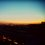Note by Boi (보이)
3 years, 8 months ago

This discussion board is a place to discuss our Daily Challenges and the math and science related to those challenges. Explanations are more than just a solution — they should explain the steps and thinking strategies that you used to obtain the solution. Comments should further the discussion of math and science.

When posting on Brilliant:

• Use the emojis to react to an explanation, whether you're congratulating a job well done , or just really confused .
• Ask specific questions about the challenge or the steps in somebody's explanation. Well-posed questions can add a lot to the discussion, but posting "I don't understand!" doesn't help anyone.
• Try to contribute something new to the discussion, whether it is an extension, generalization or other idea related to the challenge.

MarkdownAppears as
*italics* or _italics_ italics
**bold** or __bold__ bold
- bulleted- list
• bulleted
• list
1. numbered2. list
1. numbered
2. list
Note: you must add a full line of space before and after lists for them to show up correctly
paragraph 1paragraph 2

paragraph 1

paragraph 2

[example link](https://brilliant.org)example link
> This is a quote
This is a quote
    # I indented these lines
# 4 spaces, and now they show
# up as a code block.

print "hello world"
# I indented these lines
# 4 spaces, and now they show
# up as a code block.

print "hello world"
MathAppears as
Remember to wrap math in $$ ... $$ or $ ... $ to ensure proper formatting.
2 \times 3 $2 \times 3$
2^{34} $2^{34}$
a_{i-1} $a_{i-1}$
\frac{2}{3} $\frac{2}{3}$
\sqrt{2} $\sqrt{2}$
\sum_{i=1}^3 $\sum_{i=1}^3$
\sin \theta $\sin \theta$
\boxed{123} $\boxed{123}$

Sort by:

This is the answer sheet. Ask for solutions if you're curious, or you can submit your solution as well, if you want to.

To prevent any possible spoiler, I will hide it into the reply. Click on the blue button below to show the answer sheet.

- 3 years, 8 months ago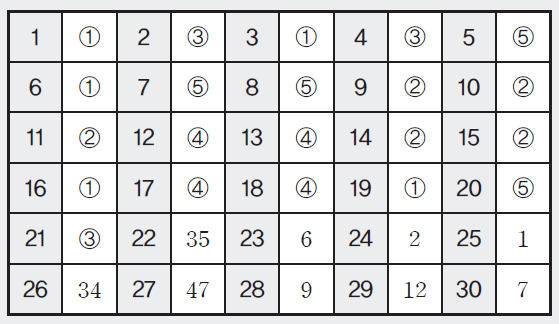(circled numbers from 1~5 are equivalent to A~E respectively.)

- 3 years, 8 months ago

These are nice questions.What would be a respectable score?What do top rankers get?

- 3 years, 6 months ago

I believe the top 4% score would be around 94~100.

- 3 years, 6 months ago

Please send solution for11 and 14

- 6 months, 2 weeks ago

For question 11, we see that the length of the square base is always (sin x - cos x). Therefore the area of the square at any given x is (sin x - cos x)^2, which is equal to 1-sin(2x), when simplified using Pythagorian and Double-Angle identities. From here we simply integrate this function from 0 to pi/6.

For question 14, since the length of BC is 2, the length of BE is half that, which is 1. Therefore, BD is also 1. (If you don't understand this part, simply draw a line from the center of the circle to E and D, and you'll see that the two right triangles are congruent, and therefore BE=BD)

Angle DBE is θ, so angle BDE can be expressed as (pi/2 - θ/2). When we apply the sine law in triangle BDE, (BE / sin BDE) = (DE / sin θ). BE is 1 and angle BDE is (pi/2 - θ/2), so let's express the sine law again: (1 / cos (θ/2)) = (DE / sin θ). Here we can multiply both sides by sin θ. This gives us: DE = sin θ / cos (θ/2).

The same applies for EF, so to calculate S(θ), we only need the angle DEF. From simple calculations we understand that angle DEF is equal to θ, therefore S(θ) equals (1/2) × DE × EF × sin θ, which is

(sin θ)^3 / {2 cos (θ/2)^2}.

When θ approaches 0, (sin θ / θ) approaches 1. cos θ/2 approaches 1 too. Therefore the value of S(θ)/θ^3 equals 1/2, giving us the answer B.

- 6 months ago

Thank you

- 5 months, 4 weeks ago

I want to refer solutions to certain questions, particularly of 3D shapes.Can you please provide the solutions to this q. paper, thankyou

- 6 months, 1 week ago

Can you please send a solution for 13?

- 4 months ago

By the central limit theorem, $\overline{X}$ ~ $N\left(m, \dfrac14\right)$, let's call it $Y$.

Normalize $X$ and $Y$ so that they both ~ $N(0, 1)$

For $X$, $Z = \dfrac{X - m}{1} = X - m$

For $Y$, $Z = \dfrac{Y - m}{1/4} = 4(Y - m)$

From the condition $P(X \geq 5) = P(Y \leq 1)$,

Change into $Z$, we have $P[Z \geq 5 - m] = P[Z \leq 4(1 - m)]$

Since the graph of $N(0, 1)$ distribution is symmetric about the origin, $5 - m = -4(1 - m) \implies m = \dfrac95.$

- 3 months ago

Hi, I think your answer for question 30 should be 5 instead of 7. Am I right?

- 1 year, 3 months ago

Nope

- 12 months ago

shouldn't answer for q20 be 3? f'(x) is always coming greater than pi/2 in the interval

- 2 months, 1 week ago

$f'(x)=-\frac{\pi}{2}(e^{x}-1)\sin(\frac{\pi}{2}x)+e^{x}\cos(\frac{\pi}{2}x)+\frac{\pi}{2}$

$f'(0)=1+\frac{\pi}{2}$ and $f'(1)=\frac{\pi}{2}(2-e)$.

From the Intermediate Value Theorem there must exist a value for b in the open interval $(0,1)$ that satisfies $f'(b)=0$.

- 2 months, 1 week ago